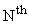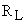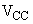Time: 3 Hours                                                                                                     Max. Marks: 100

NOTE: There are 9 Questions in all.

·      Question 1 is compulsory and carries 20 marks. Answer to Q. 1. must be written in the space provided for it in the answer book supplied and nowhere else.

·      Out of the remaining EIGHT Questions answer any FIVE Questions. Each question carries 16 marks.

·      Any required data not explicitly given, may be suitably assumed and stated.

Q.1 A.        Choose the correct or best alternative in the following:                                    (2x10)

a.       The unity gain bandwidth of 741 OPAMP is typically

(A)  4 MHz.                                        (B)  2 MHz.

(C)    6 MHz.                                       (D)  1 MHz.

b.   The conversion time of a dual-slope ADC is typically in the range of

(A)    5 to 10 ns.                                    (B)  10 to 100 ns.

(C)  100 to 200 ns.                              (D)  2 to 3 ns.

c.   In a transistor switch, the voltage change from base-to-emitter which is adequate to accomplish the switching is only about

(A)     0.2 V.                                          (B)  0.4 V.

(C)  0.1 V.                                          (D)  0.5 V.

d.   Worst case ECL noise margins are approximately

(A)     100 mV.                                      (B)  50 mV.

(C)  250 mV.                                      (D)  400 mV.

e.   A certain multiplexer can switch one of 32 data inputs to its output.  How many different inputs does this MUX have?

(A)     30 data inputs & 5 select inputs.

(B)     32 data inputs and 4 select inputs.

(C)     32 data inputs and 5 select inputs.

(D)    None of the above.

f.    What J-K input condition will always set ‘Q’ upon the occurrence of the active clock transition?

(A)    J = 0, K = 0                                 (B) J = 1, K = 1

(C) J = 1, K = 0                                  (D) J = 0, K = 1

g.   Given a MOD-14 ripple counter using J-K flip-flops.  If the clock frequency to the counter is 30 KHz, then the output frequency of the counter will be

(A) 2.2 KHz.                                       (B) 30 KHz.

(C) 2.14 KHz.                                     (D) 3.2 KHz.

Q.1 B.   State True or False

h.   The amplifiers in the sample and hold circuit are used to provide voltage amplification.

(A)   True                                             (B)  False

i.   In a Chebyshev filter of odd order, the oscillatory curve of the magnitude

response does not start from unity

(A) True                                              (B)  False

j.    The bit storage cells in a RAM, when high speed is required make use of a BJT

(A)   True                                              (B)  False

Answer any FIVE Questions out of EIGHT Questions.

Each question carries 16 marks.

Q.2     a.   Which are the important building blocks in the architecture of the 741-type OPAMP?  Comment on the function of each block.                         (8)

b.   Define the following for an OPAMP

(i)  Input bias current                            (ii)  Input offset voltage

Briefly describe how the input offset voltage can be measured.                            (6)

c.   The IC 741 OPAMP has a slew rate of 0.5V per microsecond.  If this is to be used at a frequency of 5.31 KHz, find the maximum undistortion sine wave amplitude.                                                   (2)

Q.3     a.   What are the advantages of an active filter?  Draw the circuit of a second order Low-pass active filter and explain its functioning.                          (11)

b.   Design a unity gain Low-pass active filter to meet the following specifications:

Roll off rate = - 40 dB/decade

Passband as flat as possible

Cut off frequency = 2 KHz,

dc gain = 5.                                                                                                       (5)

Q.4     a.   Write the equation for the squared magnitude response of anorder Chebyshev filter with equiripple passband and monotonic stopband and explain the typical magnitude response characteristic for the above filter.                                                    (8)

b.   Explain how a transistor can be used as a switch to connect and disconnect a loadfrom the source.                                                                                                                (8)

Q.5     a.   What is the advantage of using Schottky transistors in a TTL gate with totempole output?  Draw the circuit of a 2-input Schottky TTL gate and explain its features?                                                         (12)

Q.6     a.   What is a full adder?  Write the schematic and truth table of a full adder.  Describe how a full adder can be implemented using EX-OR/OR/AND gates.                                                                      (11)

b.   What do you mean by a data selector?  Draw the logic circuit for a two-input multiplexer using basic gates and briefly explain its operation.           (5)

Q.7     a.   What is an ADC?  Draw the schematic of an ADC that uses a binary counter and explain its operation.                                                                  (10)

b.   The parameters of a counter type ADC are given below:

Clock frequency = 1 MHz,

Comparator threshold = 0.1 mV,

Full scale output of DAC used = 10.23,

DAC input = 10-bits,

Find the following:

(i)                  The digital equivalent obtained for an analog input of         3.728 V.

(ii)                The conversion time.

(iii)               The resolution of the converter.                                                  (6)

Q.8     a.   What is a flip-flop (FF)?  What are the other names by which it is known?  How many outputs a flip-flop has and what are they called?  What are the two types of inputs does a clocked FF have?        (7)

b.   What is a shift register?  With a neat schematic explain how J-K flip-flops can be arranged to operate as a four-bit shift register.                            (9)

Q.9     a.   How are digital circuits employing MOSFETS categorised?  Define each one of them and mention their important features.  How does CMOS internal circuitry differ from N-MOS?      (8)

b.   What is meant by of the term RAM?  What is its meaning?  How is it used in computers and what is its major disadvantage?  Distinguish between a Static RAM and a Dynamic RAM.                            (8)